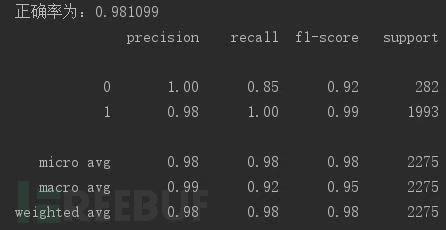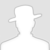FreeBuf.COM网络安全行业门户，每日发布专业的安全资讯、技术剖析。FreeBuf+小程序

2018-11-21 13:22:47

## 基于机器学习的攻击检测系统

### 问题

• 攻击的特征如何进行的提取？

• 攻击数据集的获取

• 采用什么样的算法进行训练？

### 前期准备

`字词的重要性随着它在文件中出现的次数成正比增加，但同时会随着它在语料库中出现的频率成反比下降。`

### 复现

```def split_url(data_set,num = 3):
data_str= []
for s in data_set:
s = s.strip()
s = " ".join([s[i:i+num] for i in range(len(s)-2)])
data_str.append(s)
return data_str
```

```# 逻辑回归
# 98.9%
# lr = LogisticRegression()
# lr.fit(x_train,y_train)
# predictions = lr.predict(x_test)
# pre = lr
```
```#朴素贝叶斯
# 准确率为：98.3%
nb = MultinomialNB()
nb.fit(x_train,y_train)
predictions = nb.predict(x_test)
```
```# knn
# 准确率为：94.8%   n = 6 数据集 50000 速度很慢
ner = KNeighborsClassifier(n_neighbors=6).fit(x_train,y_train)
predictions = ner.predict(x_test)
pre = ner
```

### 再现

#### 1、SQL注入检测```# 朴素贝叶斯
# 97.3%
# 逻辑回归
# 98 %
# knn
# 97.9%   n = 6
```

#### 2、实现多类型的攻击检测

1、TD-IF特征，机器学习算法实现

```def parse_data(file_path):
data_set = []
#读取数据并且进行分割，保存
with open(file_path) as f:
for s in lines_list:
if s.startswith("GET") or s.startswith("POST"):
s = s.split()[30:]
s = re.split(r"[/.?%&=+]",s)
s = " ".join(s)
data_set.append(s)
print(len(data_set))
np.save("normal_traffic.npy",data_set)
```

```publico/anadir.jsp?id=2&nombre=Jam%F3n+Ib%E9rico&precio=85&cantidad=%27%3B+DROP+TABLE+usuarios%3B+SELECT+*+FROM+datos+WHERE+nombre+LIKE+%27%25&B1=A%F1adir+al+carrito
```

```publico anadir jsp id 2 nombre Jam F3n Ib E9rico precio 85 cantidad  27 3B DROP TABLE usuarios 3B SELECT * FROM datos WHERE nombre LIKE  27 25 B1 A F1adir al carrito
```
```#逻辑回归
```

2、自编码特征，机器学习算法

• URL长度
• 参数部分长度
• 参数的个数
• 参数的最大长度
• 参数的数字个数
• 参数值中数字所占比例
• 参数值中字母所占比例
• 特殊字符个数
• 特殊字符所占比例
• 相邻路径间的编辑距离

```for i in range(len(data_link)):
per_fea = []
per_fea.append(url_len)
if len(s) != 1:
par_len = len(s)
par = s.split("&")
par_num = len(par)
par_max_l = 0
number_num = 0
str_num = 0
spe_num = 0
for pa in par:
[par_name,par_val] = pa.split("=")
if par_max_l < len(par_val):
par_max_l = len(par_val)
# pdb.set_trace()
number_num = number_num + len(num_regex.findall(par_val))
str_num = str_num + len(zimu_regex.findall(par_val))
spe_num = len(par_val) - len(num_regex.findall(par_val)) - len(zimu_regex.findall(par_val))
number_rt =  number_num / len(par_val)
str_rt = str_num / len(par_val)
spe_rt = spe_num / len(par_val)
```

```array([18.        , 33.        ,  1.        , 24.        ,  0.        ,
0.        , 23.        ,  0.95833333,  1.        ,  0.04166667])
array([22.        , 69.        ,  5.        , 16.        ,  3.        ,
0.5       , 32.        ,  5.33333333,  0.        ,  0.        ])
```

```# 逻辑回归
# 正确率：73%
# 朴素贝叶斯
# 准确率：50.2%
# svm
# 正确率为：92.1%  ，速度较慢
# knn
# 正确率：90.9%   n = 6
```

3、卷积神经网络

```#初始化权重
def init_weight(shape,std_dev):
weight = tf.Variable(tf.truncated_normal(shape,stddev= std_dev))
return weight
#初始化偏置
def init_bias(shape,std_dev):
bias = tf.Variable(tf.truncated_normal(shape,stddev=std_dev))
return bias
#定义卷积层
def conv_2d(x,w):

#池化层
def max_pool_2x2(x):
#全连接层
def fully_connected(input_layer,weights,biases):
return tf.nn.tanh(layer)
```
```#卷积模型
#第一层
shape_w = [10,3,1,32]
shape_b = 
weight_1 = init_weight(shape_w,std_dev=0.01)
bias_1 = init_bias(shape_b,std_dev=0.01)
```

```# # 对数据进行降维
# pca = PCA(n_components=0.98)
# pca.fit(data)
# data_set = pca.transform(data)
```

### 红日AI小组分析工具- 页面展示[^1]: 用机器学习玩转恶意URL检测 https://www.freebuf.com/articles/network/131279.html

# 机器学习

+ 收入我的专辑• 0 文章数
• 0 评论数
• 0 关注者